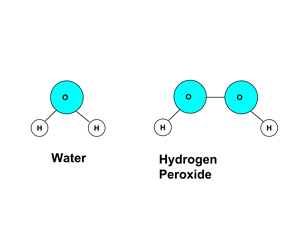# Enduring Understanding 1.A: Atoms

• All matter is composed of tiny indivisible particles called atoms.
• This is called the "Atomic Theory of Matter"
• Atoms exist in a limited number of specific types, called elements.
• There are less than one hundred different elements that occur in nature. Another 15 or so have been made in very small quantities in laboratories.
• The different elements are listed in the Periodic Table of the Elements.
• Examples of elements: hydrogen (H), oxygen (O), neon (Ne), gold (Au).
• Large samples of a given number of atoms of the same natural element (i.e. samples containing many millions of individual atoms) will have a constant mass.
• In chemistry, we often use the quantity the 'mole' when discussing very large quantities of atoms. The mole is defined as (6.02 x 1023) atoms or particles, a number called "Avogadro's number". The 'molar mass' is the mass of one mole of atoms or molecules, often expressed in grams per mole ('g/mol')
• Example: One mole (6.02 x 1023) of atoms of hydrogen (H) will always have a mass of 1.008 g, and one mole of oxygen (O) atoms will always have a mass of 15.999 g. This is called the atomic weight or molar mass.
• Atoms of the same and different types can combine together to form molecules. Molecules that contain more than one type of atom are called compounds.
• Molecules are composed of atoms in specific combinations and proportions. Sometimes, the same elements can combine in more than one proportion.
• Example: Two atoms of hydrogen (H) and one of oxygen (O) combine to form the compound H2O, water. Two atoms of hydrogen (H) and two of oxygen (O) combine to form the compound H2O2, hydrogen peroxide. H2O and H2O2 are different molecules, with different chemical and physical properties.
• <• Because (1) the average mass of any large number of atoms of an element is always the same for that element, and (2) a given compound is always composed of a combination of atoms in a specific ratio...
• Therefore, the ratio of the masses of the elements in a pure sample of a compound is always the same.
• Example 1: Water, H2O, is composed of 2 hydrogens (2 x 1.01 g/mol, or 2.02 g/mol) and one oxygen (15.999 g/mol), and therefore has a molar mass of 18.02 g/mol. Its mass composition is (2.02/18.02) or 11.2% hydrogen and (15.999/18.02) or 88.8% oxygen.
• Example 2: Hydrogen peroxide, H2O2, is composed of 2 hydrogens (2 x 1.01 g/mol, or 2.02 g/mol) and two oxygens (2x15.999 g/mol, or 31.998 g/mol), and therefore has a molar mass of 34.018 g/mol. Its mass composition is (2.02/34.018) or 5.9% hydrogen and (31.998/34.018) or 94.1% oxygen.
• So, if we are told that a pure compound of hydrogen and oxygen has a composition of about 11% hydrogen and 89% oxygen, we can conclude that the compound is water, and not hydrogen peroxide.

• The molecular formula is the number of atoms of each element in a single molecule of a substance.

• The empirical formula is the lowest whole number ratio of elements in a compound.
• In the example above, the molecular formula of hydrogen peroxide is H2O2, but the empirical formula of hydrogen peroxide is HO.

• Therefore, for any sample of a pure substance, there is a numerical relationship between the mass of the sample, the molar mass of the elements in the sample, and the ratio of the elements present in a molecule of the substance.

• Example 3: How many moles of H3PO4 has the same number of oxygen atoms as 0.5 moles of Ca(OH)2?
• There are two O atoms in each mole of Ca(OH)2, so there are 1.0 moles of O atoms. H3PO4 has four O atoms per mole, so there would be 1.0 moles of O atoms in 1.0/4 or 0.25 moles of H3PO4.

 Related Links: Chemistry Chemistry Quizzes AP Chemistry Notes

To link to this Atoms page, copy the following code to your site: